JEE  >  Test: Previous Year Questions: Binomial Numbers

# Test: Previous Year Questions: Binomial Numbers

Test Description

## 14 Questions MCQ Test Mathematics (Maths) Class 11 | Test: Previous Year Questions: Binomial Numbers

Test: Previous Year Questions: Binomial Numbers for JEE 2023 is part of Mathematics (Maths) Class 11 preparation. The Test: Previous Year Questions: Binomial Numbers questions and answers have been prepared according to the JEE exam syllabus.The Test: Previous Year Questions: Binomial Numbers MCQs are made for JEE 2023 Exam. Find important definitions, questions, notes, meanings, examples, exercises, MCQs and online tests for Test: Previous Year Questions: Binomial Numbers below.
Solutions of Test: Previous Year Questions: Binomial Numbers questions in English are available as part of our Mathematics (Maths) Class 11 for JEE & Test: Previous Year Questions: Binomial Numbers solutions in Hindi for Mathematics (Maths) Class 11 course. Download more important topics, notes, lectures and mock test series for JEE Exam by signing up for free. Attempt Test: Previous Year Questions: Binomial Numbers | 14 questions in 14 minutes | Mock test for JEE preparation | Free important questions MCQ to study Mathematics (Maths) Class 11 for JEE Exam | Download free PDF with solutions
 1 Crore+ students have signed up on EduRev. Have you?
Test: Previous Year Questions: Binomial Numbers - Question 1

### If in the expansion of (1 + x)m (1 – x)n, the co-efficients of x and x2 are 3 and – 6 respectively, then m is [JEE 99,2 ]

Detailed Solution for Test: Previous Year Questions: Binomial Numbers - Question 1

(1+x)m (1−x)n = (1 + mx + m(m−1)x2/2!)(1 − nx + n(n−1)x2/2! )
= 1 + (m−n)x + [(n2 − n)/2 − mn + (m2 − m)/2] x2
Given m − n = 3 or n = m − 3
Hence (n2 − n)/2 − mn + (m2 − m)/2 = 6
⇒ [(m−3)(m−4)]/2 − m(m−3) + (m2 − m)/2 = −6
⇒ m2 − 7m + 12 − 2m2 + 6m + m2 − m + 12 = 0
⇒ − 2m + 24 = 0
⇒ m = 12.

Test: Previous Year Questions: Binomial Numbers - Question 2

### For 2 £ r £ n,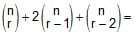Test: Previous Year Questions: Binomial Numbers - Question 3

### Find the largest co-efficient in the expansion of (1 + x)n, given that the sum of co-efficients of the terms in its expansion is 4096.     [REE 2000 (Mains)]

Detailed Solution for Test: Previous Year Questions: Binomial Numbers - Question 3

We know that, the coefficients in a binomial expansion is obtained by replacing each variable by unit in the given expression.
Therefore, sum of the coefficients in (a+b)^n
= 4096=(1+1)n
⇒ 4096=(2)n
⇒ (2)12=(2)n
⇒ n=12
Here n is even, so the greatest coefficient is nCn/2  i.e., 12C6

Test: Previous Year Questions: Binomial Numbers - Question 4

In the binomial expansion of (a - b)n, n ³ 5, the sum of the 5th and 6th terms is zero. Then equals.

Detailed Solution for Test: Previous Year Questions: Binomial Numbers - Question 4

It is given that T6​+T5​=0.
Hence nC4an−4b4−nC5an−5b5=0
nC4​an−4b4= nC5an−5b5
nC4​a = nC5b
n!b/(n−4)!4! = n!b/(n−5)!5!
= a/(n−4) = b/5​
Therefore a/b=(n−4)/5

Test: Previous Year Questions: Binomial Numbers - Question 5

Find the coefficient of x49 in the polynomial [REE 2001 (Mains), 3]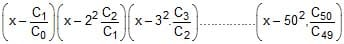where Cr = 50Cr

Test: Previous Year Questions: Binomial Numbers - Question 6

The sum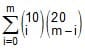, (where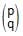= O if P < q) is maximum when m is [JEE 2002 (Scr.), 3]

Detailed Solution for Test: Previous Year Questions: Binomial Numbers - Question 6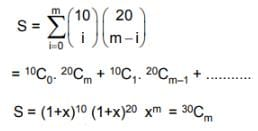Test: Previous Year Questions: Binomial Numbers - Question 7

(a) Coefficient of t24 in the expansion of (1 + t2)12 (1 + t12) (1 + t24) is [JEE 2003 (Scr.), 3]

(b) Prove that :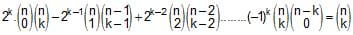[JEE 2003 (Mains),2]

Detailed Solution for Test: Previous Year Questions: Binomial Numbers - Question 7

(1+x)12 (1+x12) (1+x24)
= [C0 + C1x2 + C2x4 + C3x6 + C4x8 +.......C12x24) (1 + x12 + x24 + x36)
= x24(12C0 + 12C6 + 12C12)
= 1 + 12C6 + 1
= 12C6  + 2

Test: Previous Year Questions: Binomial Numbers - Question 8

n_1Cr = (k2 – 3). nCr + 1if k Î       [JEE 2004 (Scr.)]

Detailed Solution for Test: Previous Year Questions: Binomial Numbers - Question 8

Formula,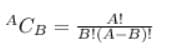(n - 1)!/{r! × (n - 1 - r)!} = (k² - 3) × n!/(r + 1)!(n - r - 1)!
or, (n - 1)!/r! = (k² - 3) × n!/(r + 1)!
or, (n - 1)!/r! = (k² - 3) × n(n - 1)!/(r + 1)r!
or, 1/1 = (k² - 3) × n/(r + 1)
or, (r + 1)/n = (k² - 3)
we know, r and n are integers so, (r + 1)/n  (0, 1]
So, 0 < (r + 1)/n ≤ 1
or, 0 < k² - 3 ≤ 1
or, 3 < k² ≤ 4
or, √3 < k ≤ 2 , -2 ≤ k < -√3
Hence, k  (√3, 2]

Test: Previous Year Questions: Binomial Numbers - Question 9

n-1Cr = (k2 - 3). nCr + 1 [JEE 2004 (Scr.)]

Detailed Solution for Test: Previous Year Questions: Binomial Numbers - Question 9

(n - 1)!/{r! × (n - 1 - r)!} = (k² - 3) × n!/(r + 1)!(n - r - 1)!
or, (n - 1)!/r! = (k² - 3) × n!/(r + 1)!
or, (n - 1)!/r! = (k² - 3) × n(n - 1)!/(r + 1)r!
or, 1/1 = (k² - 3) × n/(r + 1)
or, (r + 1)/n = (k² - 3)
we know, r and n are integers so, (r + 1)/n  (0, 1]
so, 0 < (r + 1)/n ≤ 1
or, 0 < k² - 3 ≤ 1
or, 3 < k² ≤ 4
or, √3 < k ≤ 2 , -2 ≤ k < -√3
hence, k  (√3, 2]

Test: Previous Year Questions: Binomial Numbers - Question 10

The value of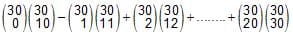is, where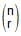= nCr        [JEE 2005 (Scr.)]

Detailed Solution for Test: Previous Year Questions: Binomial Numbers - Question 10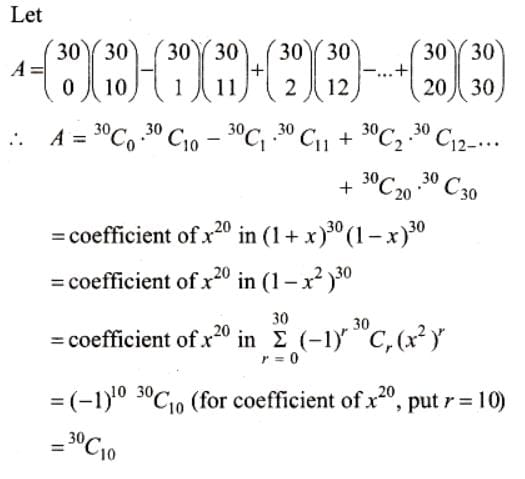Test: Previous Year Questions: Binomial Numbers - Question 11

The number of seven digit integers, with sum of the digits equal to 10 and formed by using the digits 1, 2 and 3 only, is               [JEE 2009]

Test: Previous Year Questions: Binomial Numbers - Question 12

For r = 0, 1, ...., 10 let Ar, Br, Cr denote, respectively, the coefficient of xr in the expansions of (1 + x)10, (1 + x)20 and (1 + x)30. Then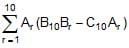is equal to       [JEE 2010]

Test: Previous Year Questions: Binomial Numbers - Question 13

Let an denote the number of all n-digit positive integers formed by the digits 0, 1 or both such that no consecutive digits in them are 0. Let bn = the number of such n-digit integers ending with digit 1 and cn = the number of such n-digit integers ending with digit 0.         [JEE 2012]

Which of the following is correct ?

Test: Previous Year Questions: Binomial Numbers - Question 14

Let an denote the number of all n-digit positive integers formed by the digits 0, 1 or both such that no consecutive digits in them are 0. Let bn = the number of such n-digit integers ending with digit 1 and cn = the number of such n-digit integers ending with digit 0.                 [JEE 2012]

The value of b6 is

## Mathematics (Maths) Class 11

156 videos|176 docs|132 tests
 Use Code STAYHOME200 and get INR 200 additional OFF Use Coupon Code
Information about Test: Previous Year Questions: Binomial Numbers Page
In this test you can find the Exam questions for Test: Previous Year Questions: Binomial Numbers solved & explained in the simplest way possible. Besides giving Questions and answers for Test: Previous Year Questions: Binomial Numbers, EduRev gives you an ample number of Online tests for practice

## Mathematics (Maths) Class 11

156 videos|176 docs|132 tests